A New Numerical Method of Estimates of Temperatures along a Thick Steel Slab and Concentrations of Alcohol along a Hollow Tube | OMICS International
Journal of Applied & Computational Mathematics

# A New Numerical Method of Estimates of Temperatures along a Thick Steel Slab and Concentrations of Alcohol along a Hollow Tube

Odekunle MR1 and Babuba S2*

1Department of Mathematics, Modibbo Adama University of Technology, Yola, Nigeria

2Department of Mathematics, Federal University Dutse, Jigawa, Nigeria

*Corresponding Author:
Sunday Babuba
Department of Mathematics
Federal University Dutse
Jigawa, Nigeria
Tel: +234 807 079 3965
E-mail: [email protected]

Received Date: June 25, 2017; Accepted Date: July 22, 2017; Published Date: July 28, 2017

Citation: Odekunle MR, Babuba S (2017) A New Numerical Method of Estimates of Temperatures along a Thick Steel Slab and Concentrations of Alcohol along a Hollow Tube. J Appl Computat Math 6: 355. doi: 10.4172/2168-9679.1000355

Copyright: © 2017 Odekunle MR, et al. This is an open-access article distributed under the terms of the Creative Commons Attribution License, which permits unrestricted use, distribution, and reproduction in any medium, provided the original author and source are credited.

Visit for more related articles at Journal of Applied & Computational Mathematics

#### Abstract

A new continuous numerical method based on the approximation of polynomials is here proposed for solving the equation arising from heat transfer along a thick steel slab and a hollow tube subject to initial and boundary conditions. The method results from discretization of the heat equation which leads to the production of a system of algebraic equations. By solving the system of algebraic equations we obtain the problem approximate solutions.

#### Keywords

Polynomials; Interpolation; Multistep collocation; Heat conduction

#### Introduction

The development of numerical techniques for solving heat conduction equation in science and engineering subject to initial and boundary conditions is a subject of considerable interest. In this paper, we develop a new continuous numerical method which is based on interpolation and collocation at some point along the coordinates (Odekunle, 2008). To do this we let U(x,t) represents the temperature at any point in the slab and the tube. Heat is flowing from one end to another under the influence of the temperature gradient ∂U/∂x. To make a balance of the rate of heat flow in and out of the media, we consider R for thermal conductivity of the steel, C the heat capacity which we assume constants, and ρ the density and D the thermal diffusivity of alcohol . Heat flow in the slab is given by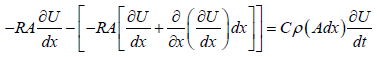(1.0)

Heat flow through the tube is also given by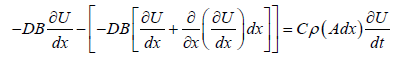(1.1)

Where A and B are the cross sections of the slab and the tube respectively.

The new method strives to provide solutions to the heat flow eqns. (1.0) and (1.1).

#### Solution Method

To set up the solution method we select an integer N such that N>0. We subdivide the interval 0 ≤ x ≤ x into N equal subintervals with mesh points along space axis given by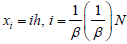, where Nh=X, similarly, we reverse the roles of x and t and we select another integer M such that M > 0. We also subdivide the interval 0 ≤ t ≤ T into M equal subintervals with mesh points along the time axis given by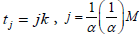where MK=T and h,k are the mesh sizes along space and time axes, respectively . Here, we seek for the approximate solution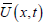to U(x,t) of the form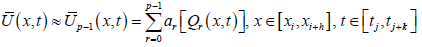(2.0)

Over h > 0, k > 0 mesh sizes, such that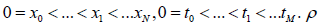is the sum of interpolation points along the space and time coordinates. That is ρ = g + b, where g is the number of interpolation points along the space axis and b the number of interpolation points along time coordinate. The basis function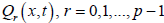is the Taylor’s polynomials which is known, ar are the constants to be determined. There will be flexibility in the choice of the basis function as may be desired for specific application. For this work, we consider the Taylor’s polynomials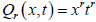[3-7].

The interpolation values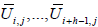are assumed to have been determined from previous steps, while the method seeks to obtain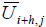(Odekunle, 2008). Applying the above interpolation conditions on eqn. (2.0) we obtain,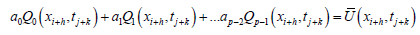(2.1)

We let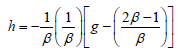arbitrarily and k=0, then by Crammer’s rule, eqn. (2.1) becomes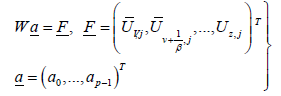(2.2)

and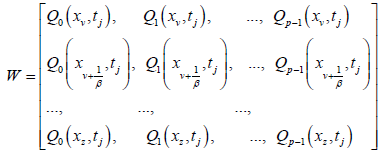Where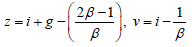and W-1 exists (Odekunle, 2008]). Hence, by equation (2.2) we obtain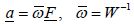(2.3)

The vector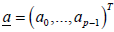is now determined in terms of known parameters in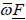. If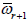is the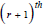row of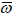then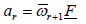(2.4)

Eqn. (2.4) determines the values [8-17]. Let us take first and second derivatives of eqn. (2.0) with respect tox,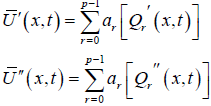(2.5)

Substituting eqn. (2.4) into eqn. (2.5), we obtain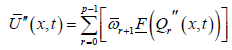(2.6)

We reverse the roles of x and t in eqn. (2.1) and we arbitrarily set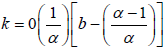and h=0, by Crammer‘s rule eqn. (2.1) becomes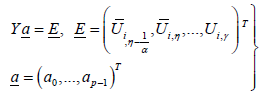(2.7)

and [18-20]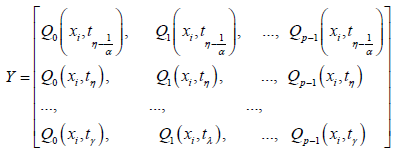Where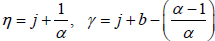, and Y-1 exists (Odekunle, 2008). Hence,

from eqn. (2.7) we obtain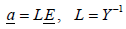(2.8)

The vector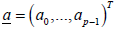is now determined in terms of known parameters in LE. [21-23]. If Lr+1 is therow ofthen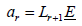(2.9)

Also, eqn. (2.9) determines the values of ar. Taking the first derivatives of eqn. (2.0) with respect to t, we obtain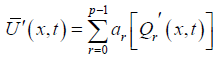(2.10)

Substituting eqn. (2.9) in eqn. (2.10) we have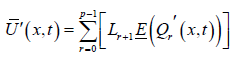(2.11)

But by eqn. (1.0) or (1.1) it is obvious that eqn. (2.11) is equal to eqn. (2.6), therefore,(2.12)

Collocating eqn. (2.12) at x=xi and t=tj we obtain a new numerical scheme that solves eqns. (1.0) and (1.1) explicitly.

#### Numerical Examples

In this section we give some numerical examples to compute approximate solutions for equations (1.0) and (1.1) by the method discussed in this paper . This is in order to test the numerical accuracy of the new method. To achieve this, we truncate the Taylor’s polynomial after second degree and use it as the basis function in the computation. The resultant scheme is used to solve the following two problems.

Example 1 (Eyaya, 2010)

Given a 2 cm thick steel slab, solve for the temperatures as a function of x and t at t=2.062 seconds if the initial temperatures are given by the relation [24-26].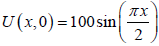where k for steel is 0.13 cal/sec °cm, c=0.11 cal/g°C and p=7.8 g/cm3.

Solution

By simplification eqn. (1.0) becomes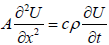. To solve this equation we take Δx=0.25 cm, then we find Δt by the relation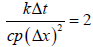, Δt=0.825 sec.We let β=4, α=64 arbitrarily which implies that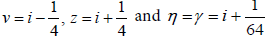. Taking two interpolation points along space coordinates and one along time implies that g=2,b=1,p=3 and for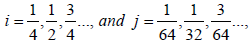we obtain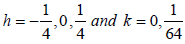, then the calculated temperatures are tabulated as shown in Table 1 .

T x=0 x=0.25 X=0.50 x=0.75 x=1.00 x=1.25
0.0 0.0 38.27 70.71 92.39 100 92.39
0.825 0.0 37.54 69.37 90.63 98.10 90.63
1.65 0.0 36.83 68.05 88.91 96.23 88.91
2.475 0.0 36.13 66.76 87.23 94.40 87.23
3.3 0.0 35.45 65.49 85.59 92.61 85.59
4.125 0.0 34.77 64.24 83.94 90.85 83.94

Table 1: Calculated temperatures.

Example 2 (Eyaya, 2010)

A hollow tube 25 cm long is initially filled with air containing 2% of ethyl alcohol vapors. At the bottom of the tube is a pool of alcohol which evaporates in to the stagnant gas above . (Heat transfers to the alcohol from the surroundings to maintain a constant temperature of, 30°C at which temperature the vapor pressure is 0.1 atm.). At the upper end of the tube, the alcohol vapors dissipate to the outside air, so the concentration is essentially zero. Considering only the effects of molecular diffusion, determine the concentration of alcohol as a function of time and distance measured from the top of the tube.

Solution

Molecular diffusion follows the law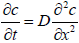where D is the diffusion coefficient, with units in cm2/sec. (This is the same as for the ratio k/cp which is often termed thermal diffusivity). For ethyl alcohol D=0.111 cm2/sec at 30°c, and the vapor pressure is such that 10 volume percent alcohol in air is present at the surface . The initial condition is c(x,0)=3.0 and the boundary conditions are given by c(0,t)=0, c(25,t)=15. Since the length of the tube is 25 cm, we take Δx=5 cm using the maximum value permitted for Δt yields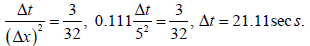If we take β=4 , α=3 arbitrarily then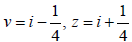and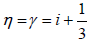. Taking two interpolation points along space coordinates and one along time implies that g=2, b=1, implies that p=3 and for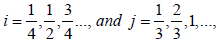we obtain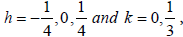then the calculated concentrations of alcohol are tabulated as shown in Table 2.

t x=0 x=5 x=10 x=15 x=20 x=25
0 0 3.00 3.00 3.00 3.00 15
21.11 0 2.63 3.00 3.00 4.50 15
42.22 0 2.35 2.95 3.19 5.63 15
63.33 0 2.13 2.91 3.47 6.50 15
84.44 0 1.96 2.88 3.78 7.18 15
105.55 0 1.83 2.88 4.09 7.73 15
126.66 0 1.73 2.90 4.39 8.18 15
147.77 0 1.66 2.94 4.68 8.56 15
168.88 0 1.61 2.99 4.95 8.88 15

Table 2: Concentrations of alcohol.

#### References

Select your language of interest to view the total content in your interested language

### Article Usage

• Total views: 768
• [From(publication date):
July-2017 - Dec 06, 2019]
• Breakdown by view type
• HTML page views : 678Can't read the image? click here to refresh